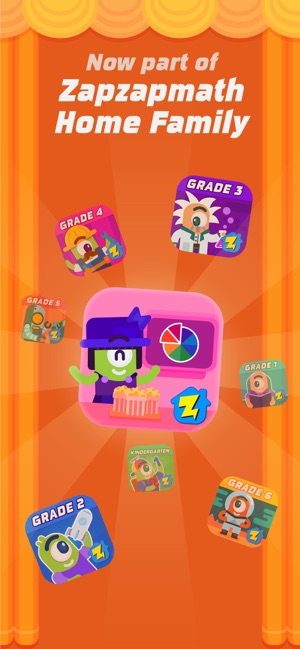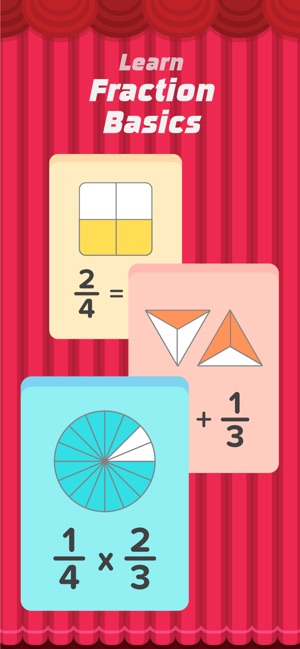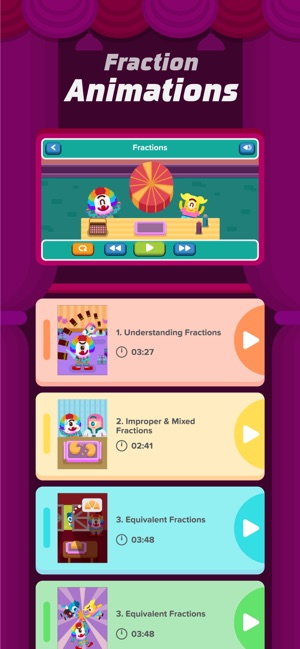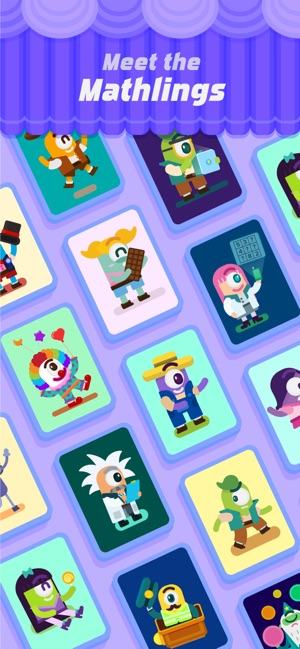## Screenshots

•••••## Description

Fraction Basics is now part of the Zapzapmath Home family!

This video app teaches you the core concepts of fractions in 12 animated videos featuring Zapzapmath's Mathlings.

This video app is divided into the following parts listed below to help you build a strong foundation in fractions:

1. Understanding Fractions
• Introduction
• Numerator, denominator of a fraction & proper fractions
• The meaning of fractions
• Improper fractions & mixed fractions

2. Improper & Mixed Fractions
• Introduction
• Proper fractions & improper fractions
• Convert an improper fraction to a mixed fraction
• Convert mixed fractions to improper fractions

3. Equivalent Fractions
• What are equivalent fractions?
• Examine equivalent fractions visually (by multiplication)
• Reasoning behind equivalent fractions
• Can we get equivalent fractions by addition or subtraction?

• Introduction: Quick recap on fractions
• Basics behind adding fractions with like denominators
• Basics behind adding fractions with unlike denominators
• Example on adding fractions with unlike denominators

5. Adding Fractions Examples
• Example 1: Adding a whole number with a proper fraction
• Quick way of adding for Example 1 above
• Example 2: Adding a mixed fraction with a proper fraction (Like Denominators)
• Example 3: Adding a mixed fraction with a proper fraction (Unlike Denominators)

6. Subtracting Fractions
• Introduction: The idea behind subtracting fractions
• Visually examine how the subtraction works
• Example 1: Subtracting two fractions with unlike denominators
• Example 2: Subtracting a proper fraction with a mixed fraction

7. Multiplying Fractions
• Introduction
• Example 1: Multiplying two proper fractions
• Examine how multiplying fractions work in Example 1
• Example 2: Multiplying two fractions & simplifying the result

8. Multiplying Fractions Examples
• Introduction
• Example 1: Simplify before multiplying two proper fractions
• Example 2: More on simplifying fractions before multiplying
• Example 3: Multiplying two mixed fractions

9. Dividing Fractions
• Introduction
• Example 1: Dividing two proper fractions
• Examine the ideas behind dividing fractions in Example 1
• Example 2: Another example on dividing fractions

10. Dividing Fractions Examples
• Example 1: Dividing two proper fractions
• Example 2: Dividing a proper fraction with a mixed fraction
• Converting the mixed fraction to an improper fraction before dividing
• Example 3: Dividing two mixed fractions

11. Convert Fraction to Percent
• Introduction
• Converting two fifths to percent
• Examine the percentage when the numerator is increased
• A quicker way of converting two fifths to percent

12. Convert Decimal to Fraction
• Introduction
• Analyze the fraction, one half
• Examples

ZAPZAPMATH HOME ALL ACCESS PASS
► Unlock all Fraction Videos with your All Access Pass
► Go beyond Fractions - your All Access Pass unlocks all content for Zapzapmath Home grades K through 6 too!

Fraction Basics is brought to you by ZapZapMath, a trusted name in educational games.

ZAPZAPMATH’S SUBSCRIPTION TERMS AND CONDITIONS
► Up to three accounts per subscription

Your subscription will be automatically renewed unless auto-renewal is disabled at least 24 hours before the subscription expires.

Renewals cost the same as the original subscription, and payment will be charged to your iTunes account upon renewal confirmation.

You may turn off auto-renewal at any time after purchase by going to your iTunes Account Settings, but refunds will not be given for any unused portion of the term.

The price above is for U.S. customers. Pricing in other countries may vary, and charges may be converted to your local currency. Please refer to our:

Version 3.1.4

- Fixed bugs.

## Ratings and Reviews

1.0 out of 5
1 Rating

1 Rating

LorFa ,

### Excellent!!!!

Best app ever. Sometimes fractions are so hard to understand but this app explain things so easy, basics and much more! Now my son will excel at fractions thanks to this app. Love it.

Ahmed drdrt ,

### Ahmed

Best app ever serious it will make you better than a nerd without being one

GamingTurtle_69 ,

### Awesome !

Perfect app for learning fractions! :)

Helped pass my Fractions test :)

## Information

Seller
Visual Math Interactive Sdn Bhd
Size
180.7 MB
Category
Education
Compatibility

Languages

English, Ukrainian

Age Rating
Rated 4+
© Visual Math Interactive
Price
Free
In-App Purchases
1. Zapzapmath Home - Monthly \$7.99
2. Zapzapmath Home - Yearly \$62.99

## Supports

•